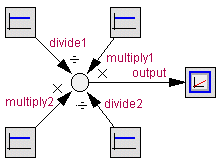﻿ 20-sim webhelp > Library > Signal > Block Diagram > multiplydivide

# multiplydivide

## Library

Signal\Block Diagram , System

## Use

Domains: Continuous, Discrete. Size: [n,m]. Kind: Block Diagrams.

## Description

This model yields a multiplication and/or division of one or more input signals. While connecting input signals, 20-sim will ask whether the signals should be multiplied or divided. The signals may have either size, but the size of all connected signals should be equal.For example the MultiplyDivide model can have 2 signals connected that are multiplied and 2 signals that must be divided. 20-sim treats them as an array multiply and an array divide with:

multiply = [multiply1;multiply2];

and

divide = [divide1;divide2];

The output will then be equal to:

output = (multiply1 * multiply2) / (divide1 * divide2);

## Interface

 Inputs Description multiply[any] divide[any] Any number of input signals (each of the same size) may be connected to be multiplied (multiply) or divided (divide). Outputs output

## Limitations

The signals connected to this model should all have the same size.